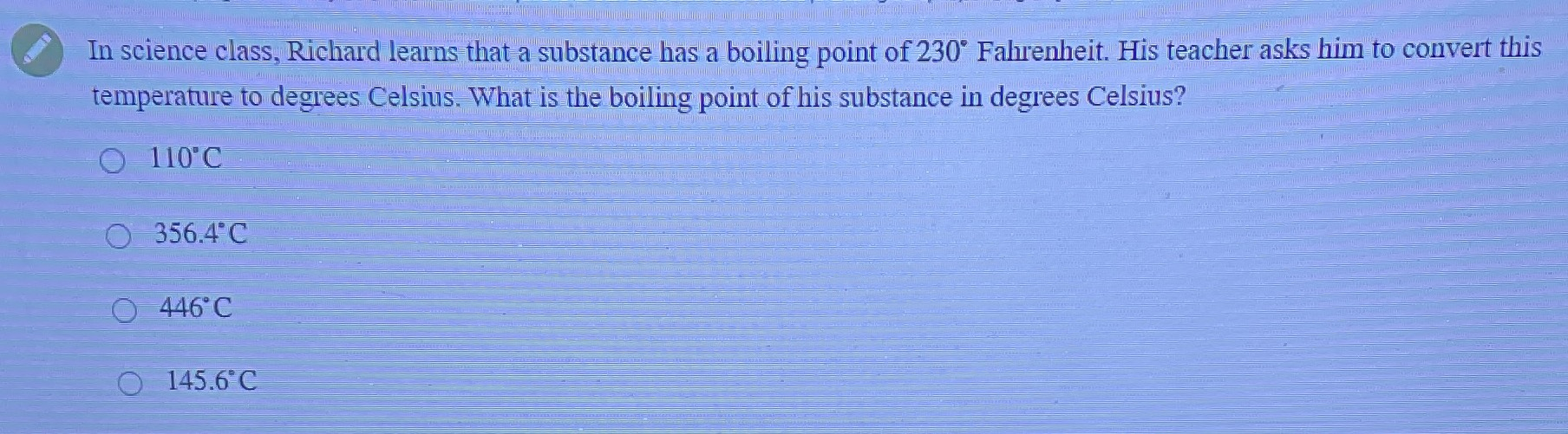### Still have math questions?

Algebra
Question(In science class, Richard learns that a substance has a boiling point of $$230 ^ { \circ }$$ Fahrenheit. His teacher asks him to convert this temperature to degrees Celsius. What is the boiling point of his substance in degrees Celsius?

$$110 ^ { \circ } C$$

$$356.4 ^ { \circ } C$$

$$446 ^ { \circ } C$$

$$145.6 ^ { \circ } C$$